# Lecture 2: An Introduction to Number Theory

In this lecture, we are going to discuss the first properties in the field of number theory. These are essential for our continued study of cryptology. We can do little more without some basic definitions and results. Whenever possible, examples will be used to illustrate the ideas. The hope is that this brief introduction inspires the reader to study this beautiful field more thoroughly.

### Prime Numbers

A positive integer is called a prime number if it is divisible only by one and itself. Primes form the building blocks of all numbers because of the following theorem.

Theorem [Fundamental Theorem of Arithmetic]: Every positive integer is uniquely a product of prime numbers.

Of course, given a number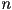, a prime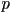can appear on its list more than once. For instance, the numberMuch later on, we will see that factoring a number into its prime factors is extremely difficult and plays an important role in cryptology. But first, we continue to study our prime numbers.

We know that there are infinitely many numbers. And we know that each can be written uniquely as a product of primes. So it seems that there must be quite a few prime numbers. However, is it possible that only finitely many prime numbers could generate all of the numbers? The answer to this question goes back to Euclid.

Theorem: There are infinitely many prime numbers.

Proof: Suppose that there were only finitely many prime numbers. Then, we could write them all down in a list,. Consider now the number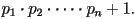It is necessarily larger than any of the primes on our list, and so therefore is not prime. But, from the Fundamental Theorem of Arithmetic, it has a prime factorization. This means, in particular, that it is divisible by one of the primes on our list. This cannot be the case however, since our number divided by any prime on our list give remainder 1. Thus, our list is incomplete and there are infinitely many primes.

### Greatest Common Divisors

Letand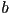. be positive integers. By the Fundamental Theorem of Arithmetic, bothandhave factorizations into products of prime numbers. We would like a way to measure how much the factorizations overlap. Fortunately, this is easy. The greatest common divisor ofand(or) is largest positive integer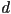such thatdivides bothand.

For example,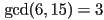. We will see in a moment that greatest common divisors exist for any two positive integersand. Ifandare such that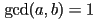, then we say thatandare relatively prime. For example, 8 and 15 are relatively prime. This terminology comes from the fact that they have no common proper divisor other than 1; much like how a prime number has no proper divisor other than 1.

In particular, ifis prime, then we know thatis a divisor offor any. Thus we have that. The latter case only occurs whenis a multiple of.

Proposition: Given two positive integersand,exists and is unique.

As we mentioned above, factorization is a very difficult process, so while the above proof shows how to construct the greatest common divisor, it is not very efficient. In the next section, we will discuss an algorithm which works much better.

### The Division Algorithm

Given two positive integers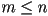, we can do grade school long division to find a quotientand a remainder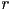so that we can write

``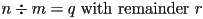''.

Reversing this procedure shows that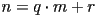. Moreover, the long division makes sure that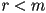, so thatandwe find are unique. Amazingly, this is all we need to do to find.

Algorithm[The Division Algorithm]: Letbe positive integers.

Then, for the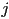where Step 4 stops, we have that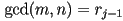.

Let's find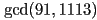. We follow the algorithm exactly:Since, we see that. Indeed, one can also check that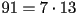and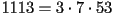.

### Modular Arithmetic

Recall from the last lecture that when we were assigning numbers to represent letters or symbols, we had to 'wrap around' once we got to the end. Accordingly, when we used the shift cipher, sometimes we had to subtract off to make sure that our ciphertext actually made sense. This is idea behind modular arithmetic: we fix a number, the modulus, and do arithmetic 'wrapping around' whenever we get bigger than.

Fix our modulus to be 38. Then if we want to compute 19+25 'wrapping around' 38, we simply find 19+25=44. Since 44>38, we need to subtract: 44-38=6. Thus, 19+25=6 'wrapped around' 38. Other operations work as well:

Let's now rigorously define how to do modular arithmetic, so that we no longer need to write 'wrapped around' each time we use it! Fix a positive integer. Then we will say integersandare congruent moduloifdivides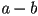. In this case, we write. We now state a theorem which explains how usual arithmetic behaves modulo.

Theorem: Letbe a positive integer, and let,, andbe any integers.

Let's re-work the previous example in our new notation.

Fix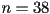Now, let's go over a few tips for actually doing modular arithmetic. First, if we are working modulo, then every number must be congruent to one of, and none of these numbers are congruent modulo(why?). Thus, we can automatically reduce any number to something less than our modulus. For example, if we are working modulo 42, we can think of 156 as 30. Or, putting it in notation,. Off the bat, this may not seem like much of a difference, but the above theorem shows that you can either do modular arithmetic first and then reduce or reduce first and then do modular arithmetic. Either way will give you the same answer! In general, it is faster to reduce first, because it makes the numbers smaller. Let's do some examples.

These are chosen to illustrate why one should reduce first, and then do the arithmetic.

### Exercises

This work was made possible through a grant from the National Science Foundation.